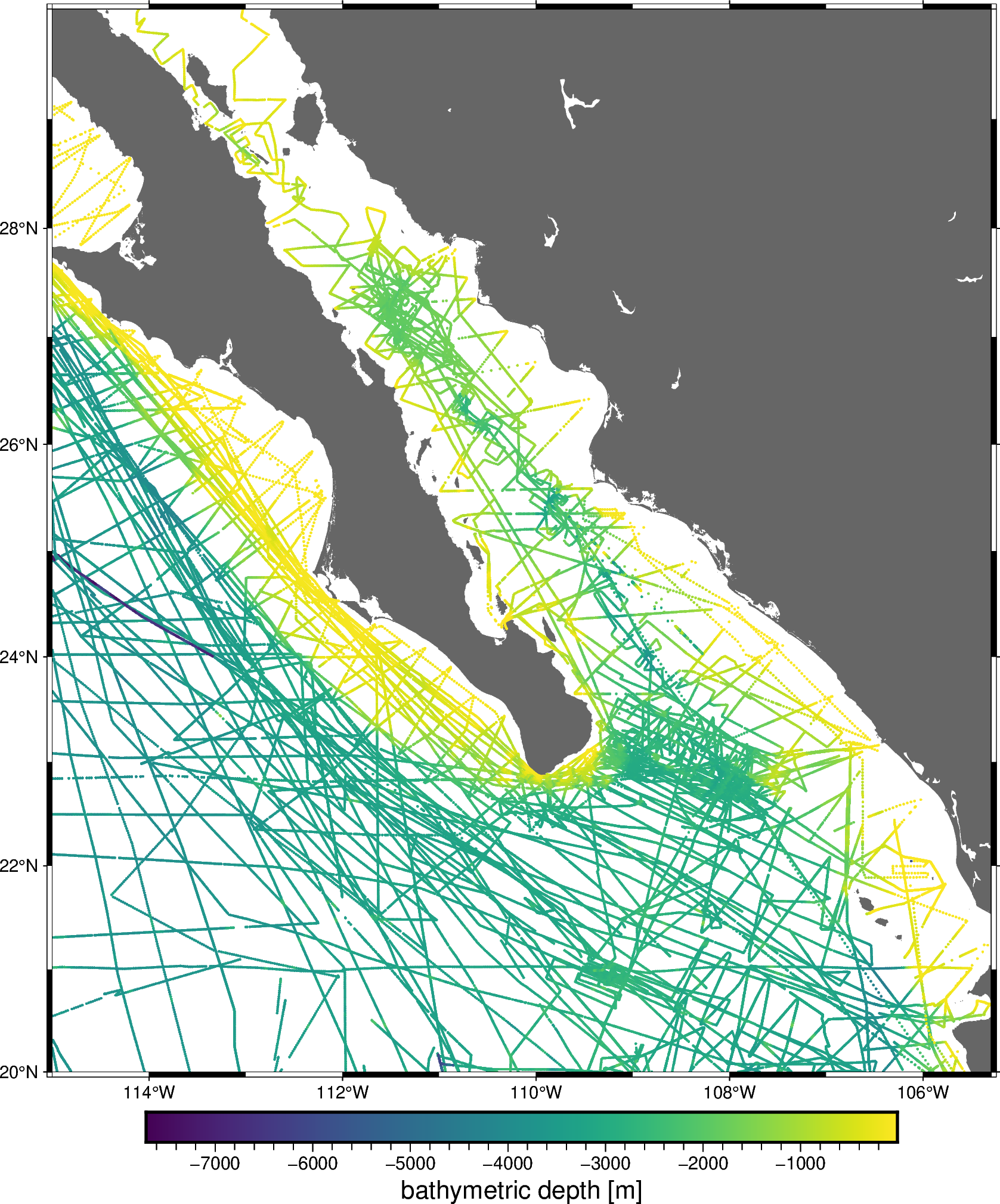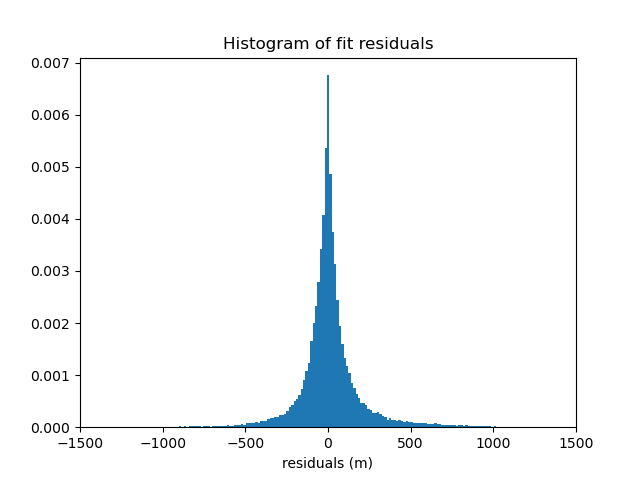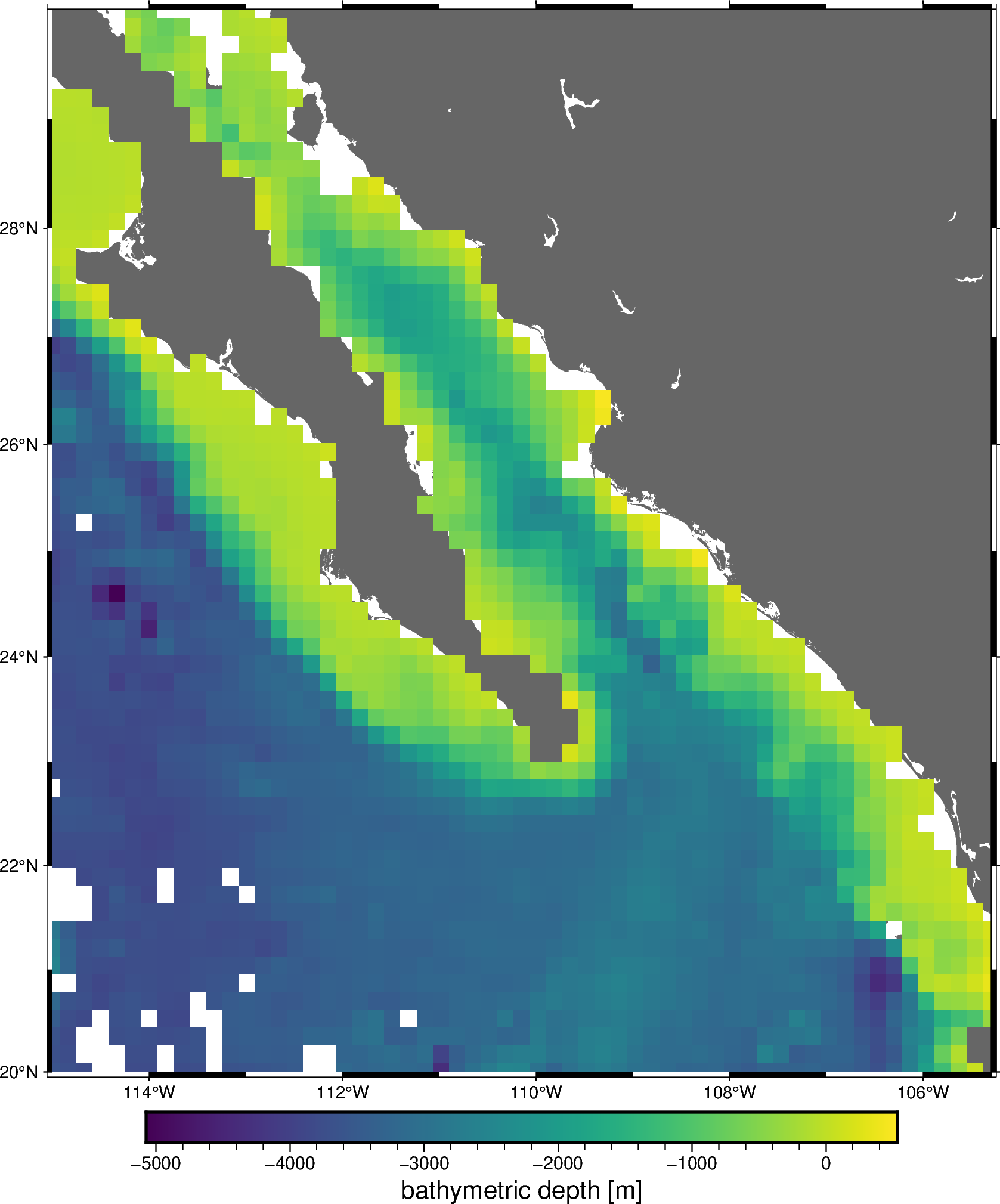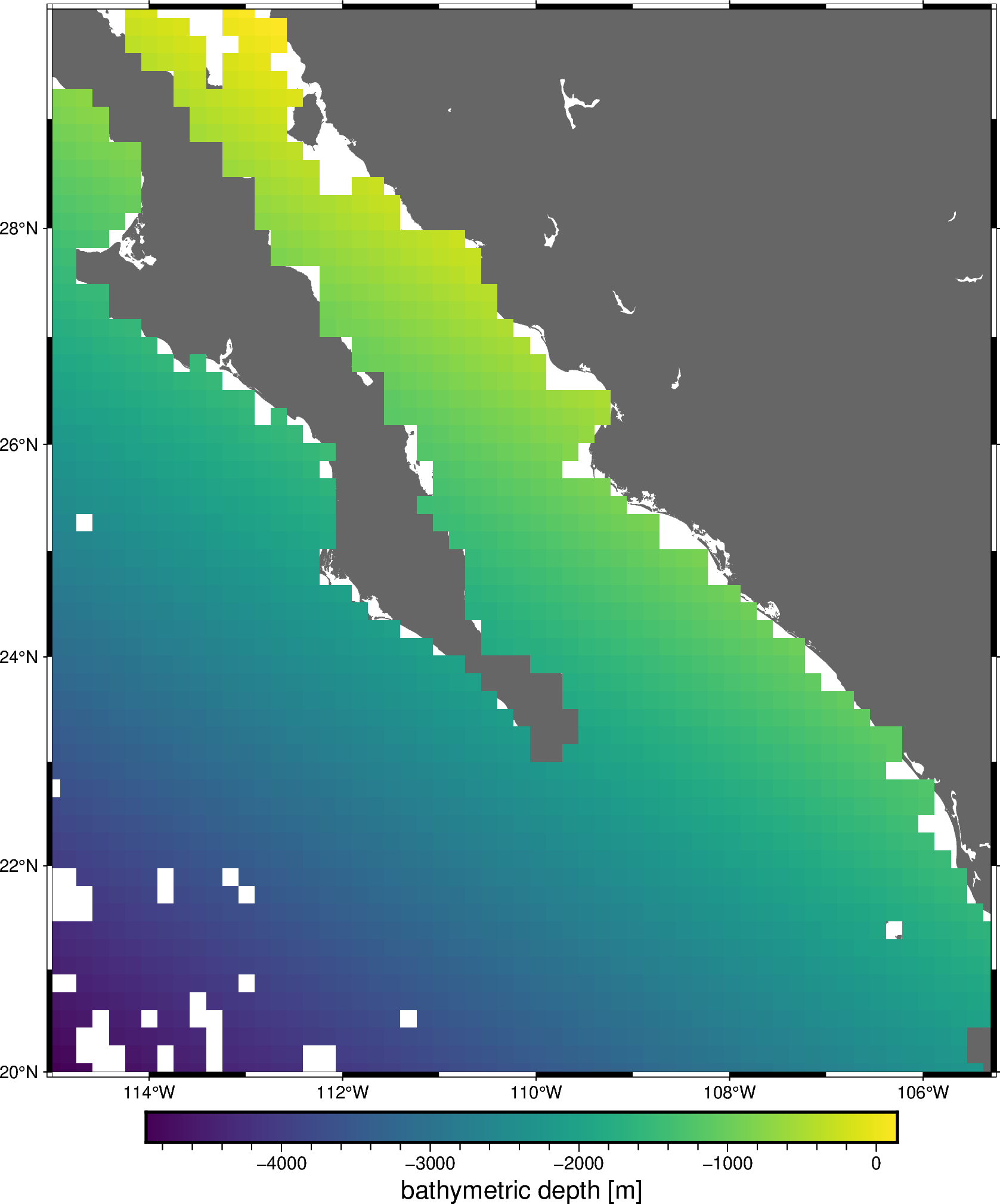# Chaining Operations#

Often, a data processing pipeline looks like the following:

1. Apply a blocked mean or median to the data

2. Remove a trend from the blocked data

3. Fit a spline to the residual of the trend

4. Grid using the spline and restore the trend

The `verde.Chain` class allows us to created gridders that perform multiple operations on data. Each step in the chain filters the input and passes the result along to the next step. For gridders and trend estimators, filtering means fitting the model and passing along the residuals (input data minus predicted data). When predicting data, the predictions of each step are added together.

Other operations, like `verde.BlockReduce` and `verde.BlockMean` change the input data values and the coordinates but don’t impact the predictions because they don’t implement the `predict` method.

Note

The `Chain` class was inspired by the `sklearn.pipeline.Pipeline` class, which doesn’t serve our purposes because it only affects the feature matrix, not what we would call data (the target vector).

For example, let’s create a pipeline to grid our sample bathymetry data.

```import matplotlib.pyplot as plt
import numpy as np
import pygmt
import pyproj

import verde as vd

data = vd.datasets.fetch_baja_bathymetry()
region = vd.get_region((data.longitude, data.latitude))
# The desired grid spacing in degrees
spacing = 10 / 60
# Use Mercator projection because Spline is a Cartesian gridder
projection = pyproj.Proj(proj="merc", lat_ts=data.latitude.mean())
proj_coords = projection(data.longitude.values, data.latitude.values)

fig = pygmt.Figure()
fig.coast(region=region, projection="M20c", land="#666666")
pygmt.makecpt(cmap="viridis", series=[data.bathymetry_m.min(), data.bathymetry_m.max()])
fig.plot(
x=data.longitude,
y=data.latitude,
color=data.bathymetry_m,
cmap=True,
style="c0.05c",
)
fig.basemap(frame=True)
fig.colorbar(frame='af+l"bathymetric depth [m]"')
fig.show()
```Out:

```<IPython.core.display.Image object>
```

We’ll create a chain that applies a blocked median to the data, fits a polynomial trend, and then fits a standard gridder to the trend residuals.

```chain = vd.Chain(
[
("reduce", vd.BlockReduce(np.median, spacing * 111e3)),
("trend", vd.Trend(degree=1)),
("spline", vd.Spline()),
]
)
print(chain)
```

Out:

```Chain(steps=[('reduce',
BlockReduce(reduction=<function median at 0x7fab4c1501f0>,
spacing=18500.0)),
('trend', Trend(degree=1)), ('spline', Spline(mindist=0))])
```

Calling `verde.Chain.fit` will automatically run the data through the chain:

1. Apply the blocked median to the input data

2. Fit a trend to the blocked data and output the residuals

3. Fit the spline to the trend residuals

```chain.fit(proj_coords, data.bathymetry_m)
```
```Chain(steps=[('reduce',
BlockReduce(reduction=<function median at 0x7fab4c1501f0>,
spacing=18500.0)),
('trend', Trend(degree=1)), ('spline', Spline(mindist=0))])```
In a Jupyter environment, please rerun this cell to show the HTML representation or trust the notebook.

Now that the data has been through the chain, calling `verde.Chain.predict` will sum the results of every step in the chain that has a `predict` method. In our case, that will be only the `Trend` and `Spline`.

We can verify the quality of the fit by inspecting a histogram of the residuals with respect to the original data. Remember that our spline and trend were fit on decimated data, not the original data, so the fit won’t be perfect.

```residuals = data.bathymetry_m - chain.predict(proj_coords)

plt.figure()
plt.title("Histogram of fit residuals")
plt.hist(residuals, bins="auto", density=True)
plt.xlabel("residuals (m)")
plt.xlim(-1500, 1500)
plt.show()
```Likewise, `verde.Chain.grid` creates a grid of the combined trend and spline predictions. This is equivalent to a remove-compute-restore procedure that should be familiar to the geodesists among us.

```grid = chain.grid(
region=region,
spacing=spacing,
projection=projection,
dims=["latitude", "longitude"],
data_names="bathymetry",
)
data_coordinates=(data.longitude, data.latitude),
maxdist=spacing * 111e3,
grid=grid,
projection=projection,
)
grid
```
```<xarray.Dataset>
Dimensions:     (latitude: 61, longitude: 59)
Coordinates:
* longitude   (longitude) float64 245.0 245.2 245.3 ... 254.4 254.5 254.7
* latitude    (latitude) float64 20.0 20.17 20.33 20.5 ... 29.66 29.82 29.99
Data variables:
bathymetry  (latitude, longitude) float64 -3.621e+03 -3.709e+03 ... nan nan
Attributes:

Finally, we can plot the resulting grid:

```fig = pygmt.Figure()
fig.coast(region=region, projection="M20c", land="#666666")
fig.grdimage(
grid=grid.bathymetry,
cmap="viridis",
nan_transparent=True,
)
fig.basemap(frame=True)
fig.colorbar(frame='af+l"bathymetric depth [m]"')
fig.show()
```Out:

```<IPython.core.display.Image object>
```

Each component of the chain can be accessed separately using the `named_steps` attribute. It’s a dictionary with keys and values matching the inputs given to the `Chain`.

```print(chain.named_steps["trend"])
print(chain.named_steps["spline"])
```

Out:

```Trend(degree=1)
Spline(mindist=0)
```

All gridders and estimators in the chain have been fitted and can be used to generate grids and predictions. For example, we can get a grid of the estimated trend:

```grid_trend = chain.named_steps["trend"].grid(
region=region,
spacing=spacing,
projection=projection,
dims=["latitude", "longitude"],
data_names="bathymetry",
)
data_coordinates=(data.longitude, data.latitude),
maxdist=spacing * 111e3,
grid=grid_trend,
projection=projection,
)
grid_trend
```
```<xarray.Dataset>
Dimensions:     (latitude: 61, longitude: 59)
Coordinates:
* longitude   (longitude) float64 245.0 245.2 245.3 ... 254.4 254.5 254.7
* latitude    (latitude) float64 20.0 20.17 20.33 20.5 ... 29.66 29.82 29.99
Data variables:
bathymetry  (latitude, longitude) float64 -4.911e+03 -4.864e+03 ... nan nan
Attributes:

```fig = pygmt.Figure()
fig.coast(region=region, projection="M20c", land="#666666")
fig.grdimage(
grid=grid_trend.bathymetry,
cmap="viridis",
nan_transparent=True,
)
fig.basemap(frame=True)
fig.colorbar(frame='af+l"bathymetric depth [m]"')
fig.show()
```Out:

```<IPython.core.display.Image object>
```

Total running time of the script: ( 0 minutes 13.391 seconds)

Gallery generated by Sphinx-Gallery